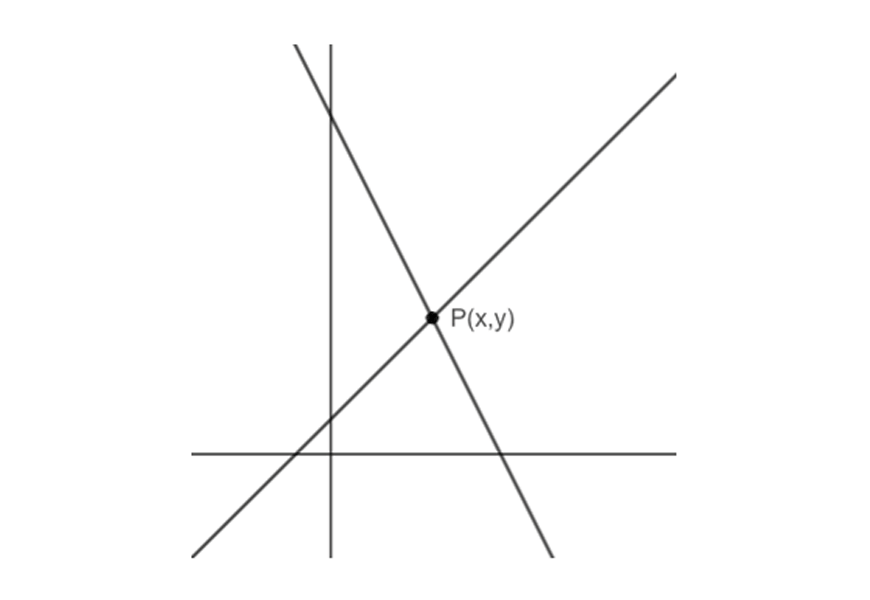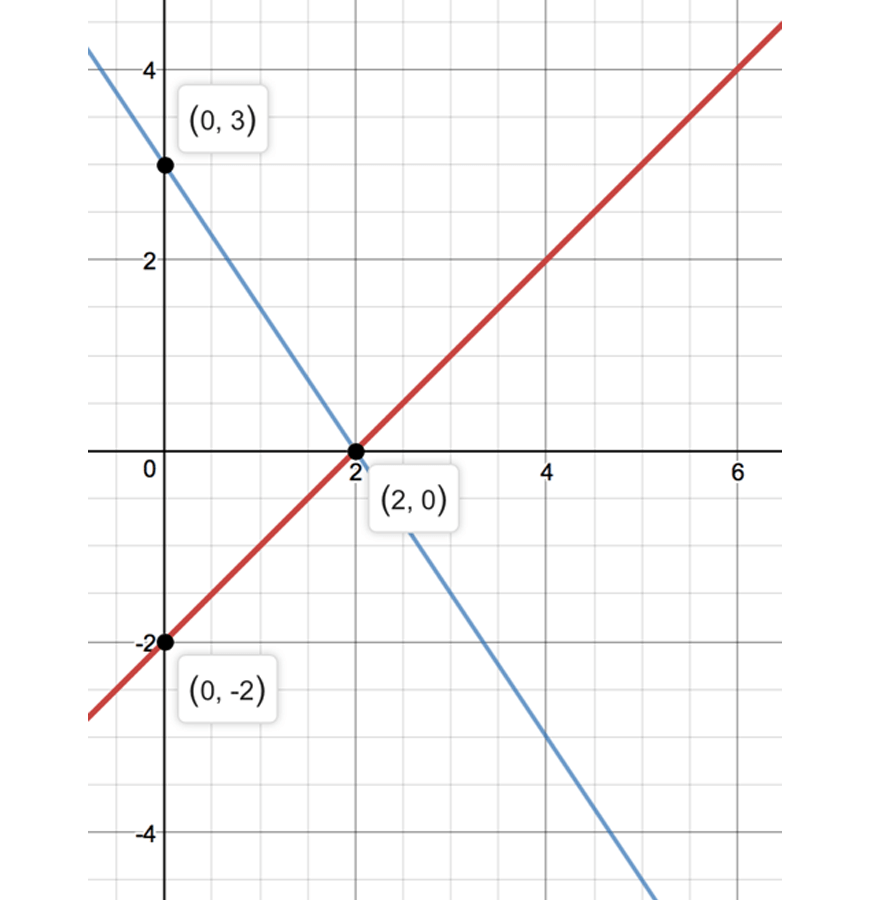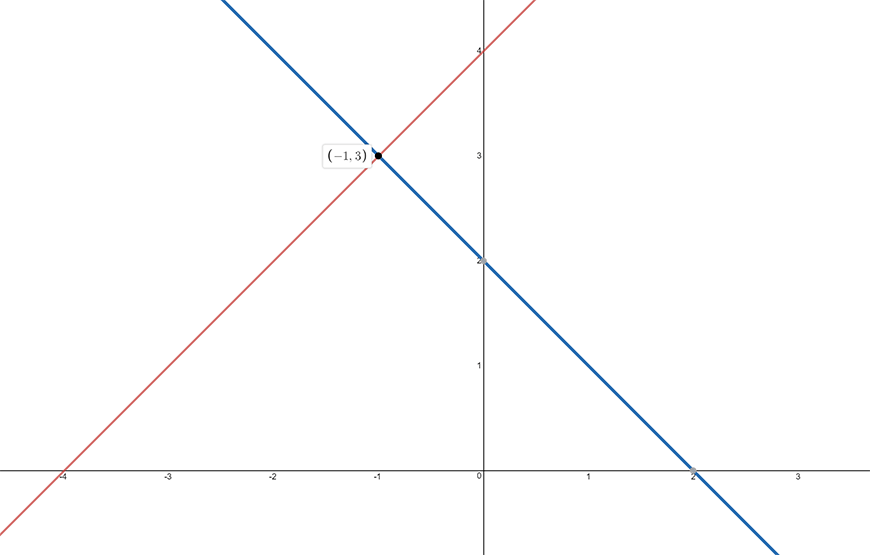# Part 7: Simultaneous Equations

In this article, we introduce you to simultaneous equations. This unit will become the foundation of your study of quadratic and non-linear equations in Year 10## Simultaneous Equations

In this Guide, we will give you a comprehensive overview and cheat-sheet to help you conquer simultaneous equations for algebra!

## NSW Syllabus Outcomes

Stage 5.3: Solves complex linear, quadratic, simple cubic and simultaneous equations and rearranges literal equations. (MAS.3-7NA)

Solve simultaneous equations, where one equation is non-linear, using algebraic and graphical techniques, including the use of digital technologies

• Use analytical methods to solve a variety of simultaneous equations where one equation is non-linear
1. Choose an appropriate method to solve a pair of simultaneous equations
• Solve pairs of simultaneous equations, where one equation is non-linear, by finding the point of intersection of their graphs using digital technologies.
1. Determine and explain that some pairs of simultaneous equations, where one equation is non-linear, may not have real solutions.

These Syllabus Outcomes are telling you that you will learn how to solve simultaneous equations algebraically and graphically.

## What’s the point of Simultaneous Equations?

Algebra is used in every field of Maths:

• Trigonometry
• Statistics
• Probability
• Vectors, etc.

In addition, algebra crops up in much of everyday life in fields like:

• Engineering
• Finance
• Medicine
• Computing
• Marketing

This article will show you the methods to solve simultaneous equations using two algebraic techniques (elimination and substitution) and graphically solve simultaneous equations.

We will also teach you to inspect the number of possible solutions to an equation without needing to solve it, by interpreting graphs.

## Assumed Knowledge

Students should be familiar with:

• Basic algebraic techniques and simple arithmetic
• Knowledge of basic curve sketching
• For harder problems, students should also know how to sketch more complicated functions such as:
• Hyperbolae
• Circles
• Semi-circles
• Exponential functions
• Cubic curves
• Note that, students will still understand the theory behind the content without knowing how to sketch these harder functions as they may instead use technology to do so.

## Simultaneous Equations

So far in your study of algebraic equations, you will have only solved linear equations that contained one pronumeral.

Consider the following diagram:The point $$P(x,y)$$ satisfies two different equations because it lies on both of the lines.

The coordinate of this point gives the unique $$x$$ and $$y$$ values that satisfy both equations simultaneously.

Thus the two equations are called a pair of simultaneous equations.

## The Graphical Method for Solving Simultaneous Equations:

We will first sketch each ‘equation’ as a line on the number plane and use these lines to find the solution to the pair of simultaneous equations.

The solution will reveal itself as the point of intersection of the two lines.

“The solution to a set of simultaneous equations is the common point of intersection”

### Example:

Solve the equations $$x-y=2$$ and $$3x+2y=6$$ simultaneously, using the graphical method.

### Solution:

First sketch the lines $$y=x-2$$ and $$y=-\frac{3}{2} x+3$$ on the number plane.We can see, graphically, that the two lines intersect at (2,0).

Thus (2,0) satisfies each equation.

Therefore, $$x=2$$ and $$y=0$$ are the solutions to the simultaneous equations.

### Limitations of the Graphical Method

The example given was very convenient – we were able to very clearly see where the two lines intersected.

But this will not always be the case, and in exam situations – we are not able to sketch the graphs with pin-point accuracy or be able to see what the solution is.

For example, solving the simultaneous equations

$$21x+5y+7=0$$ and $$2x-3y-2=0$$

has solution $$x=-\frac{11}{73}$$ and $$y=-\frac{56}{73}$$. But it would have been extremely hard to see graphically!

We must, therefore, look at some more efficient ways to solve these equations.

## Substitution Method for Solving Simultaneous Equations

Solving linear equations such as

$$x-2=\frac{(6-3x)}{2}$$

are easy because there is only one unknown pronumeral.

So when faced with a pair of simultaneous equations, we can solve for one of the unknown variables and substitute it into the other equation.

This will reduce the problem to solving a linear equation just like the one we saw.

Once we solve for the variable, we can easily solve for the other variable since we can substitute it back into either one of the original equations.

### Example:

Solve the equations $$x-y=2$$ and $$3x+2y=6$$ simultaneously using the substitution method.

### Solution:

Number the equations (so that we can talk about them easily):

$$x-y=2 … (1) \\ 3x+2y=6 … (2) \\$$

Now we make y the subject in (1)

$$y=x-2$$

and now substitute it into the other equation.

$$3x+2(x-2)=6$$

which can be solved to obtain

$$x=2$$.

We now solve for $$y$$ by substituting $$x=2$$ into either equation (it doesn’t matter!). At this point, it’s really up to you – but for this problem, it is easier to substitute it into (1):

$$2-y=2⇒y=0$$

And so

$$x=2$$ and $$y=0$$

is the solution.

## Elimination Method for Solving Simultaneous Equations

Instead of substituting, which can often lead to a lot of algebra, sometimes we can more elegantly eliminate a variable from the equations to reduce it to a linear equation.

Often elimination method may require multiplying each of the equations by a constant before either adding or subtracting the two equations to eliminate the variable.

### Example:

Solve the equations $$x-y=2$$ and $$3x+2y=6$$ simulteanously using the elimination method.

### Solution

Number the equations:

$$x-y=2 … (1) \\ 3x+2y=6 … (2) \\$$

Let’s now eliminate y, we can do this by first multiplying (1) by 2 and then adding up both of the equations.

$$2x-2y=4 … (1) \\ 3x+2y=6 … (2) \\$$

(1)+(2) gives

$$(2x-2y)+(3x+2y)=4+6 \\ 5x=10 \\ x=2 \\$$

Notice how there were no y terms once we added up the equations. This is why it is called the ‘elimination’ method.

Once we have $$x=2$$, we simply substitute it, just like before, into either equation to get $$y$$

And so

$$x=2$$ and $$y=0$$

is the solution.

With the elimination method, often students will have to be a bit more creative in order to see what constants you may have to use when adding up or subtracting the equations.

This particular method might seem hard at first – but it will really start to pay off in more complicated simultaneous equation problems.

## Summary

• If a point $$P(x,y)$$ satisfies two equations. Then $$x$$ and $$y$$ is a solution to the pair of simultaneous equations.
• Solving two equations simultaneously is equivalent to solving for the point of intersection of two graphs.

### Graphical Method

• Sketch each equation as a function, and graphically see where the solution is.
• Will not always be helpful since it can be hard to sketch accurately enough or to see what the exact values are of the solutions.

### Substitution Method

• Substitute for one of the variables in one of the equations into the other to turn the problem into solving a linear equation.

### Elimination Method

• By adding/subtracting the equations, eliminate one of the variables so that you can solve for the other variable by solving a linear equation.
• Sometimes you will need to multiply equations by a constant in order to eliminate.

### Number of Solutions

• The number of solutions to an equation is the number of points of intersections that you can see when sketching the LHS and the RHS as graphs on the same number plane.

## Concept Check Questions

### Questions

1. By graphing $$y=x+4$$ and $$y=-x+2$$ on the same number plane. Solve the equations $$x-y-4=0$$ and $$x+y-2=0$$ simultaneously.

2. Solve the equations $$x+2y-1=0$$ and $$2x+5y-2=0$$ simultaneously by using the substitution method.

3. Solve the equations $$2x-y+7=0$$ and $$2x+5y-1=0$$ simultaneously by using the elimination method.

### Solutions

1.Drawing the two lines show that $$x=-1, y=3$$  solves the pair of equations simultaneously.

2.

$$x+2y-1=0…(1) \\ 2x+5y-2=0…(2) \\$$

From (1)

$$x=1-2y$$

Substitute this into equation (2)

$$2(1-2y)+5y-2=0 \\ y=0 \\$$

When $$y=0, x=1$$

Thus $$x=1$$ and $$y=0$$ solves the equations simultaneously

3.

$$2x-y+7=0…(1) \\ 2x+5y-1=0…(2) \\ (2)-(1): 6y-8=0 \\ ∴y=\frac{4}{3} \\$$

When $$y=\frac{4}{3},x=\frac{1}{2} (\frac{4}{3}-7)=-\frac{17}{6}$$

Thus $$x=-\frac{17}{6}$$ and $$y=\frac{4}{3}$$ solves the equations simultaneously.

© Matrix Education and www.matrix.edu.au, 2019. Unauthorised use and/or duplication of this material without express and written permission from this site’s author and/or owner is strictly prohibited. Excerpts and links may be used, provided that full and clear credit is given to Matrix Education and www.matrix.edu.au with appropriate and specific direction to the original content.

### Get free study tips and resources delivered to your inbox.

Join 27,119 students who already have a head start.